# 2012 A-level H2 Mathematics (9740) Paper 2 Question 10 Suggested SolutionsAll solutions here are SUGGESTED. Mr. Teng will hold no liability for any errors. Comments are entirely personal opinions.

(i)
Firstly, gold coins are scattered randomly in a randomly chosen region of.
Secondly, the mean number of gold coins found in any randomly chosen region ofis constant.
Lastly, the finding of a gold coin in a randomly chosen region of areais independent of the finding of another gold coins.

(ii)
Let X denote the number of gold coins in a region of.(iii)
Let Y denote the number of gold coins found in a region of.Using the Graphing Calculator,(iv)
Let W denote the number of gold coins found in a region of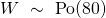Since,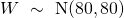approximatelyby continuity correction(v)
Let C and S denote the number of gold coins and pottery shards in in a region of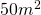respectively.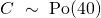Since,approximately.Since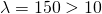,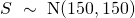approximately.approximately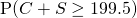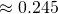(vi)approximately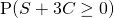by continuity correctionNot readable? Change text.